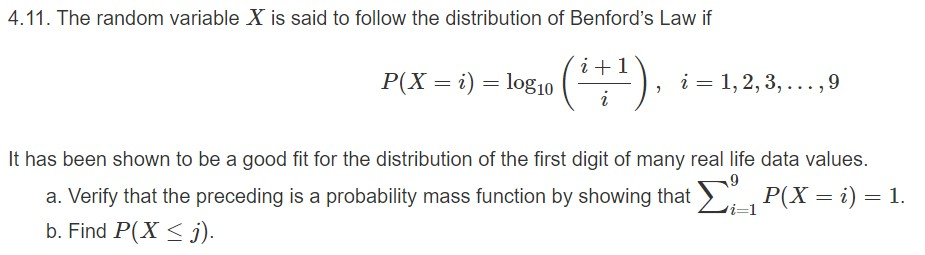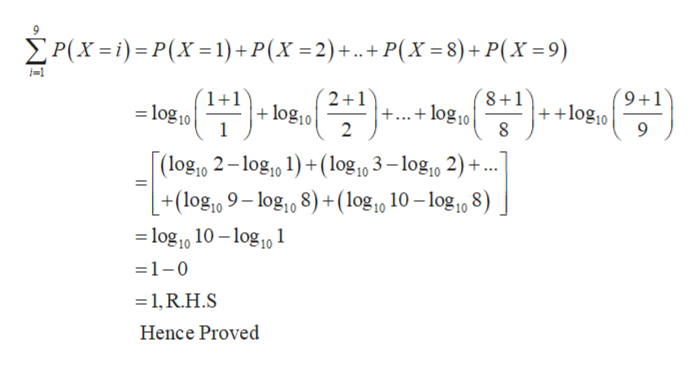# 4.11. The random variable X is said to follow the distribution of Benford's Law if(1)P(X ilog101, 2, 3,...,9iIt has been shown to be a good fit for the distribution of the first digit of many real life data values.P(X i) 1.a. Verify that the preceding is a probability mass function by showing thati-1b. Find P(Xi)

Question
23 viewshelp_outlineImage Transcriptionclose4.11. The random variable X is said to follow the distribution of Benford's Law if (1) P(X ilog10 1, 2, 3,...,9 i It has been shown to be a good fit for the distribution of the first digit of many real life data values. P(X i) 1. a. Verify that the preceding is a probability mass function by showing that i-1 b. Find P(X i) fullscreen
check_circle

Step 1

It is provided that X defined as random variable, follow the distribution of Benford’s Law.

a)

To show probability mass function, prove that

Step 2

Proof:

L.H.S...help_outlineImage TranscriptioncloseP(Xi)P(X1) +P(X =2) +.. + P(x =8)+P(X=9) 2 1 +log10 2 8+1 ++ log10 8 (9+1 +1 log.0+ +...+ log10 9 (log10 2-log10 1)(log10 3-log10 2)+.. +(log10 9-log10 8)+(log,0 10 --log10 8) -log10 10-log101 =1-0 1, R.H.S Hence Proved fullscreen

### Want to see the full answer?

See Solution

#### Want to see this answer and more?

Solutions are written by subject experts who are available 24/7. Questions are typically answered within 1 hour.*

See Solution
*Response times may vary by subject and question.
Tagged in

### Other